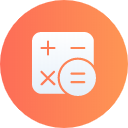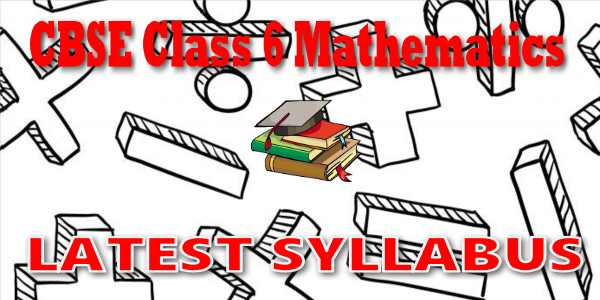No products in the cart.

# CBSE - Class 06 - Mathematics - CBSE Syllabus## CBSE, JEE, NEET, CUET

Question Bank, Mock Tests, Exam Papers

NCERT Solutions, Sample Papers, Notes, Videos

## CBSE Syllabus for Class 06 Mathematics

CBSE Syllabus Class 6 Mathematics### Student Subscription

##### Unlock the exclusive content designed for the toppers## myCBSEguide App

Complete Guide for CBSE Students

NCERT Solutions, NCERT Exemplars, Revison Notes, Free Videos, CBSE Papers, MCQ Tests & more.

# Latest CBSE Syllabus for Class 6 MathematicsCBSE syllabus for class 6 Mathematics 2018, 2019, 2020, 2021, 2022 as per cbse.nic.in new curriculum. CBSE syllabus is available for free download in PDF format. Download latest CBSE syllabus of 6th Mathematics as PDF format. Mathematics syllabus for cbse class 6 is also available in myCBSEguide app, the best app for CBSE students.

## CBSE Academics Unit - Curriculum Syllabus

CBSE has special academics unit to design curriculum and syllabus. The syllabus for CBSE class 6 Mathematics is published by cbse.nic.in Central Secondary Education, Head Office in New Delhi. The latest syllabus for class 6 Mathematics includes list of topics and chapters in Mathematics. CBSE question papers are designed as per the syllabus prescribed for current session.

## CBSE Syllabus category

• Secondary School Curriculum (class 9 and class 10)
• Senior School Curriculum (class 11 and class 12)
• Vocational Courses (Class 11 and class 12)

Course Structure for Class-VI

Number System (60 hrs)

(i). Knowing our Numbers:

Consolidating the sense of number ness up to 5 digits, Size, estimation of numbers, identifying smaller, larger, etc. Place value (recapitulation and extension), connectives: use of symbols =, >, < <,>and use of brackets, word problems on number operations involving large numbers up to a maximum of 5 digits in the answer after all operations. This would include conversions of units of length & mass (from the larger to the smaller units), estimation of outcome of number operations. Introduction to a sense of the largeness of, and initial familiarity with, large numbers up to 8 digits and approximation of large numbers)

(ii). Playing with Numbers:

Simplification of brackets, Multiples and factors, divisibility rule of 2, 3, 4, 5, 6, 8, 9, 10, and 11. (All these through observing patterns. Children would be helped in deducing some and then asked to derive some that are a combination of the basic patterns of divisibility.) Even/odd and prime/composite numbers, Co-prime numbers, prime factorization, every number can be written as products of prime factors. HCF and LCM, prime factorization and division method for HCF and LCM, the property LCM × HCF = product of two numbers. All this is to be embedded in contexts that bring out the significance and provide motivation to the child for learning these ideas.

(iii). Whole numbers

Natural numbers, whole numbers, properties of numbers (commutative, associative, distributive, additive identity, multiplicative identity), number line. Seeing patterns, identifying and formulating rules to be done by children. (As familiarity with algebra grows, the child can express the generic pattern.)

(iv). Negative Numbers and Integers

How negative numbers arise, models of negative numbers, connection to daily life, ordering of negative numbers, and representation of negative numbers on number line. Children to see patterns identify and formulate rules. What are integers, identification of integers on the number line, operation of addition and subtraction of integers, showing the operations on the number line (addition of negative integer reduces the value of the number) comparison of integers, ordering of integers.

(v). Fractions: Revision of what a fraction is, Fraction as a part of whole, Representation of fractions (pictorially and on number line), fraction as a division, proper, improper & mixed fractions, equivalent fractions, comparison of fractions, addition and subtraction of fractions (Avoid large and complicated unnecessary tasks). (Moving towards abstraction in fractions) Review of the idea of a decimal fraction, place value in the context of decimal fraction, inter conversion of fractions and decimal fractions (avoid recurring decimals at this stage), word Problems involving addition and subtraction of decimals (two operations together on money, mass, length and temperature)

Algebra (15 hrs.)

INTRODUCTION TO ALGEBRA

• Introduction to variable through patterns and through appropriate word problems and generalizations (example 5 × 1 = 5 etc.)
• Generate such patterns with more examples.
• Introduction to unknowns through examples with simple contexts (single operations)

Ratio and Proportion (15 hrs.)

• Concept of Ratio
• Proportion as equality of two ratios
• Unitary method (with only direct variation implied)
• Word problems
Geometry (65 hrs.)

(i). Basic geometrical ideas (2 -D):

Introduction to geometry. Its linkage with and reflection in everyday experience.

Line, line segment, ray.

Open and closed figures.

Interior and exterior of closed figures.

Curvilinear and linear boundaries

Angle — Vertex, arm, interior and exterior,

Triangle — vertices, sides, angles, interior and exterior, altitude and median

Quadrilateral — Sides, vertices, angles, diagonals, adjacent sides and opposite sides (only convex quadrilateral are to be discussed), interior and exterior of a quadrilateral.

Circle — Centre, radius, diameter, arc, sector, chord, segment, semicircle, circumference, interior and exterior.

(i). Understanding Elementary

Shapes (2-D and 3-D):

• Measure of Line segment
• Measure of angles
• Pair of lines – Intersecting and perpendicular lines – Parallel lines
• Types of angles- acute, obtuse, right, straight, reflex, complete and zero angle
• Classification of triangles (on the basis of sides, and of angles)
• Types of quadrilaterals – Trapezium, parallelogram, rectangle, square, rhombus.
• Simple polygons (introduction) (Up to octagons regulars as well as non-regular).
• Identification of 3-D shapes: Cubes, Cuboids, cylinder, sphere, cone, prism (triangular), pyramid (triangular and square) Identification and locating in the surroundings
• Elements of 3-D figures. (Faces, Edges and vertices)
• Nets for cube, cuboids, cylinders, cones and tetrahedrons.

(iii).Symmetry: (reflection)

• Observation and identification of 2-D symmetrical objects for reflection symmetry
• Operation of reflection (taking mirror images) of simple 2-D objects
• Recognizing reflection symmetry (identifying axes)

(iv).Constructions (using Straight edge Scale, protractor, compasses) • Drawing of a line segment

• Construction of circle
• Perpendicular bisector
• Construction of angles (using protractor)
• Angle 60°, 120° (Using Compasses)
• Angle bisector- making angles of 30°, 45°, 90° etc. (using compasses)
• Angle equal to a given angle (using compass)
• Drawing a line perpendicular to a given line from a point a) on the line b) outside the line.

## CURRICULUM / SYLLABUS OF VOCATIONAL COURSES

CURRICULUM / SYLLABUS

 Scheme of Studies and List of Vocational Courses Application form to offer vocational courses Secondary School Curriculum (Under NSQF) Senior School Curriculum (Vocational)## myCBSEguide

Trusted by 1 Crore+ Students

#### Question Paper Creator

• Create papers in minutes
• Print with your name & Logo
• 5 Lakhs+ Questions
• Solutions Included
• Based on CBSE Syllabus
• Best fit for Schools & Tutors## Test Generator

Create papers at ₹10/- per paper## CUET Mock Tests

75,000+ questions to practice only on myCBSEguide app